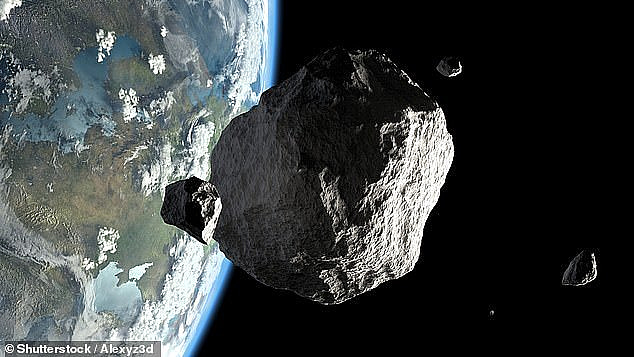# Identifying Earth-impacting asteroids using an artificial neural networkThe evolution of the orbits of planets and asteroids in the Solar system is chaotic. This means that minuscule changes in their orbit eventually leads to extraordinary variance in their positions. In the case of asteroids, this could be the difference between hitting or missing the Earth.

In the classical, Monte-Carlo, approach collision probabilities are determined by launching thousands, and even sometimes millions, of asteroids according to distributions in orbital parameters defined by the asteroids' respective observed uncertainties. The collision probability is then determined by the fraction of asteroids that strike Earth or approach with a certain minimum distance of it. When objects experience a close encounter with a planet, or when their orbits are particularly uncertain, this method fails to accurately quantize the collision probability because it does not fully explore the space of all possible outcomes; one simply cannot perform enough simulations of asteroid trajectories in order to arrive at a finite number of asteroids that would actually hit the Earth.

By performing detailed calculations of the orbits of planets and hypothetical asteroids, we acquire a library of asteroids that will collide with Earth in the near future, which we refer to as "known impactors". For this calculation, we first compute the orbits of the planets forwards in time up to 10,000 years in the future. In the next step, we then calculate the future solar system backwards in time while launching asteroids from the Earths' surface. This then creates a collection of objects that will collide with Earth once their velocities are negated. These known impactors are then used as examples of hazardous objects to train the neural network.